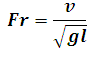# Froude Number Calculator

This Calctown Calculator calculates the froude number which is the ratio of inertial force on an element of fluid to the gravitational force.

m/s
m

#### Resultwhere

Fr = froude number

v = fluid velocity

g = acceleration due to gravity

l = mean length